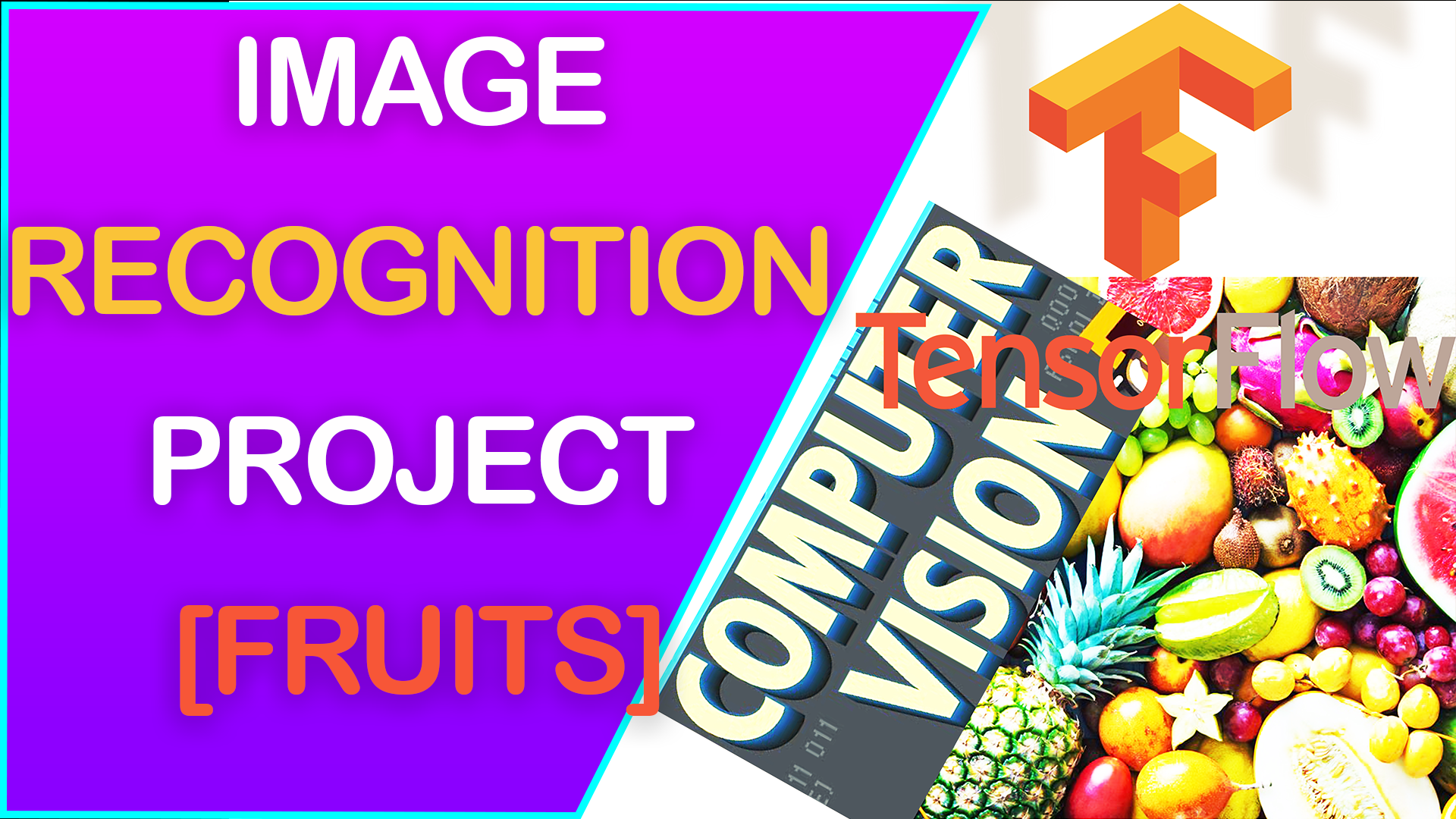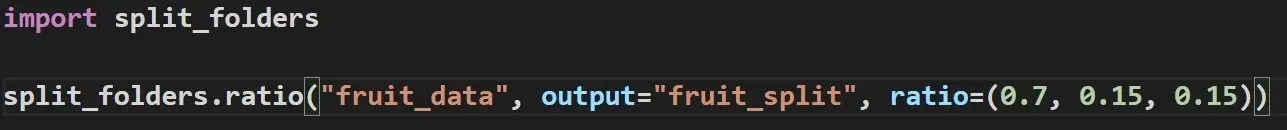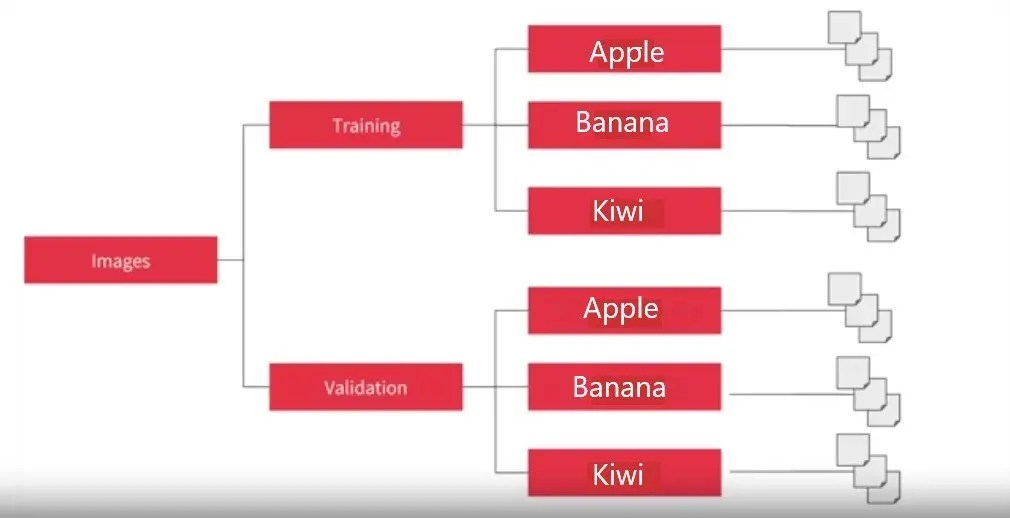# 15 Fruits Image Classification with Computer Vision and TensorFlow### Splitting Large Image Dataset into train, test, and validation datasets.

The first thing that needs to be done is to split the dataset into training, test, validation datasets.

Splilt_folders package was used to accomplish this task of splitting the image dataset into train, test, validation folder datasets without doing it manually.How to use split folders pythono package to split image dataset into training, validation, and test datasets

The initial structure of the dataset folder is:

Main Folder

Apple Folder

Apple images

Banana Folder

Banana images

Kiwi Folder

Kiwi images

E.T.C.

The desired structure of the folders for data analysis is:

Main Folder

Training Folder

Apple Folder

Apple images

Banana Folder

Banana images

Kiwi Folder

Kiwi images

E.T.C

Validation Folder

Apple Folder

Apple images

Banana Folder

Banana images

Kiwi Folder

Kiwi images

E.T.C

Test Folder

Apple Folder

Apple images

Banana Folder

Banana images

Kiwi Folder

Kiwi images

E.T.C

Here is an image of what the Image Dataset folders should look like after splitting it.What Image Dataset folders are supposed to look like before importing it into ImageDataGenerator using TensorFlow

### What libraries and modules were used in this project?

These are the libraries, packages, and modules that was used in this project including importing the OS

### The most important step is importing the image datasets by Importing training and validation dataset using ImageDataGenerator.

Importing the image datasets after it has been split was quit straight forward.

The first thing is to instantiate the ImageDataGenerator from TensorFlow which is what is used to import the images. When ImageDataGenerator is instantiated, we rescale the image from 0-255 into numbers between 0-1 because 0-255 are numbers that are too high for the model to process.

Why do we need to re-scale in the first place? To tell you why we need to re-scale in the first place, I have to tell you how a camera works.

When a photograph is taken, as the light hits the sensor in the camera, it measures the amount of red, blue, and green light contacting it and it scores them on a scale of 0-255. 255 is the largest number you can have with an 8 digit binary number. And then it puts those 3 values (red, blue, and green) into 3 cells and each group of 3 cells is 1 pixel. Pictures are made of pixels. So, an image is an array of numbers between 0-255.

The original image is all shapes and sizes, but we want the image imported to all be the same shape and size. We could do this manually, but when there are thousands of image involved. This process would be a nightmare. So, using the ImageDataGenerator we can rescale the shape of images as they are being imported, this allows for rescaling of the imported images without affecting the original image dataset. In the future, it will make it easier to change for the shape of imported images and also save us the pain of manually re-shaping thousands of images.

The class_mode was chosen to be categorical because this the dataset used in this project has multiple categories. If it has only 2 categories, then “binary” will be used as the class_mode

The train_dir is the directory that contains the folders containing 15 different folders. Each folder represent a fruit and contains the images. It is a common mistake to point the ImageDataGenerator at the individual class folders containing the images as opposed to pointing it at the training directory that contains the 15 different class folders.

After importing the image datasets, it tells you how many images are in the folder and how many different classes(categories) are there. Each class = each unique fruit.

Importing the training dataset tells us that we have 15 different fruits (classes) and over 37,000 images for training.

The validation and test dataset are also 15 different classes as expected and over 8000 images each for training and validation.

We can also take this a step further and see how much of each type of image is in each class. For example, with this line of code we can see that there are over 4000 images in the apple training dataset and over 2000 bananas in the banana training dataset.

### Previewing the list of images in The Fruit DataSet

#### It is important to print out the images and get a preview of the list of images in the folder.

These lines of code just prints a list of images in the apple and banana dataset. It only prints the first 10 images. This is one way to verify that the dataset and images was loaded correctly. It is also another way to see what the names of the images are for future reference.

#### Along side printing the list of images, it is also helpful to print an actual picture of the images to get a preview of what they look like.

What is better than printing the names of the images is actually printing the images themselves. So, in the following lines of code, the images for the  first two classes (Apples and Bananas) were printed to get a preview of what the images we are working with looks like.

### Now it is time to build the model that will be used to train the images.

To start off the model, 4 convolutional layers and 4 max pooling layers were used. And, based on the performance of this model, it will be tweaked for better image prediction performance.

Let’s take some time to explain what is happening in this model.

The first 2 convolutional layers uses a 64, 3 by 3 filter to filter the images and the last 2 convolutional layers uses 128, 3 by 3 filter.

All the maxpooling layers using a 2 by 2 mass pooling.

The activation keyword is a signal to activate that layer of neurons. Without the activation keyword, then that layer won’t be activated. Relu is one form of activation, there are many different type of activation and you can find out more about them here

As you might have noticed, the first layers specifies the input shape. This is basically telling the model network what shape the images should be in once they are imported. This input shape number should match the input shape number specified in the ImageDataGenerator.

So, what exactly is the convolutional and max pooling layers doing?

The basic idea behind a convolution is to narrow down the content of the image to focus on specific, distinct details. A filter is just an array of numbers that are passed over the image. The underlying pixels of the images will be changed based on the formula within the 3 by 3 filter matrix.

This concept of applying convolutions (filters) over images is very important in computer vision because it is the features that gets highlighted that distinguishes one item from another. So, the amount of information needed to identify something is much less because the model is training only on the highlighted features.

We do convolutions before putting images through the dense layer because then the images going into the dense layer is more focused and possibly more accurate.

The max pooling layer is designed to compress the image after it has been filtered while maintaining the highlighted features from convolution.

A 2 by 2 MaxPooling filter has the effect of creating a 2 by 2 array of pixels over the image, then picks the biggest number from the image within that 2 by 2 array. Basically, it turns 4 pixels in the image into 1. It repeats this process across the entire array that makes up the image. Doing this causes a 25% reduction in the size of the image.

Let’s go ahead and define some of the terminology used in this model.

Sequential: That defines a SEQUENCE of layers in the neural network

Flatten: Flatten takes square images (150,150) and turn them into and a 1 dimensional set of images.

Dense: Adds a layer of neurons. Each layer of neurons need an activation function to tell them what to do.

The dense layer after Flatten() is using 512 neurons, but we could also train with 1024 neurons and bigger number of neurons. Just remember that the more neurons you have, the longer it will take to do training and your accuracy may or may not improve as a result of increasing your hidden layer neurons.

Relu effectively means “If X>0 return X, else return 0” — so what it does is it only passes values 0 or greater to the next layer in the network.

Softmax takes a set of values, and effectively picks the biggest one, so, for example, if the output of the last layer looks like [0.1, 0.1, 0.05, 0.1, 9.5, 0.1, 0.05, 0.05, 0.05], it saves you from fishing through it looking for the biggest value, and turns it into [0,0,0,0,1,0,0,0,0] — The goal is to save a lot of coding!

The 15 in the last Dense layer signifies 15 different classes. This last Dense layer in the model tells us how many classes (categories) the training and validation dataset has.

### Printing the model summary will give you a preview of the process the images will go through.

After the models has been built, the summary of the model is exactly what we expect it to be.

The model tells us that it is a sequential model type. The conv2d layer shows that after convolutions, the output shape from this layer is 148 by 148 images. Remember that the original input shape is 150 by 150.

This 148 by 148 images then becomes the input of the max_pooling layer. The max pooling layer outputs 74 by 74 images which becomes the input of the next layer.

This process continues until the images passes through all the convolutional and max_pooling layers before making it to the flatten layer.

As you can see, the input of the flatten layer is from the last max_pooling layer which is a 7 by 7 by 128 3D array. When this input images is flattened, they become 1D array. That is why the output of the flatten layer is 6272. This is not surprising because 7 x 7 x 128 = 6,272

### The next thing is to compile the model.

After the model has been built, the next step is model compilation using an optimizer and loss function. What is the relationship between the loss and the optimizer?

The way neural networks work is that they start by making a guess.

The Loss Functions measures how good or bad the guess of the neural network is…AND

The Optimizer Function takes the data from the loss function and determines how good or bad the previous guess was, and then based on the data from the loss function, it figures out what the next guess is going to be.

And it continues this cycle until the network approaches 100% accuracy which is called convergence.

There are many different types of loss functions and optimizer functions . In this model, “categorical_crossentropy” was used because the dataset has multiple categories/classes. And “rmsprop” was used for the optimizer as it implements the RMSprop algorithm.

### Shortening Training Time

When I first did training with the model and images, it took 12 hours to train and validate up to 40 epochs. So, some steps were taken to shorten training time.

The steps taken to shorten training and validation times are

• Creating a callback function. This callback function will stop training when validation dataset reaches 98% accuracy.
• The other step taken to shorten training is to implement “workers” in model.fit and increase the number of workers from the default 1 to 10

### Fitting and Saving the TensorFlow Model

In the model.fit function, I specified the training dataset, the validation dataset, the number of epochs (iterations), the callback functions, and the number of workers.

When the model is done training, it has to be used to make predictions using a new image the model has not seen before. It would be a nightmare to re-train the model every time this notebook is opened in order to use it for predictions.

So, I saved the model. The model was saved using 2 different methods and basically, once the model stops training, the model will be saved. The importance of saving a model is that next time when the model wants to be used, we can just import the saved model and use it without having to re-train the model. The model was saved using .pb and .h5 method

### Graphing Loss and Accuracy Results from Model

After the model has been trained, the loss and accuracy results from the training and validation dataset was graphed to see how they progressed through out the training.

### Importing Saved Model and Evaluating It

The saved models were imported and evaluated just to verify they were correctly saved. When a saved model is imported, it has to evaluated before it can be used to make predictions. So, it was evaluated with the validation dataset because test dataset will be used to test the model.

### Using the Model to Predict Fruit Images

The next step is using the model to do predictions with images from the test dataset. The code above is how the model was used to predict the images. The test image has to be turned into an array of numbers, then pass those array of numbers into the model in order to get a prediction. Computers/Models can’t see raw images as they are, what they see/comprehend is numbers. That is why the images has to be converted to numbers first before prediction analysis.

Here is how to interpret the model predictions.

1 = What the model thinks the predicted fruit is. The models tells you its prediction by putting a 1 at the position that corresponds to that fruit.

0 = What the model predicts is NOT the fruit in question.

Positions are as follows

1 = Apple, 2 = Banana, 3 = Carambola, 4 = Guava, 5 = Kiwi, 6 = Mango, 7 = Muskmelon, 8 = Orange, 9 = Peach, 10 = Pear, 11 = Persimmon, 12 = Pitaya, 13 = Plum, 14 = Pomegranate, 15 = Tomatoes.

This means that if you upload a photo to the model and the model puts “1” in position 6 and “0” everywhere else, the model thinks the fruit you uploaded is a mango.

The model predictions are as follows:

1. Apple – Correctly Predicted as Apple
2. Banana – Correctly Predicted as Bananas
3. Carambola – Correctly Predicted as Carambola
4. Guava – Correctly Predicted as Guava
5. Kiwi – Incorrectly Predicted as Peach
6. Mango – Correctly Predicted as Mango
7. Muskmelon – Incorrectly Predicted as Peach
8. Orange – Incorrectly Predicted as Muskmelon
9. Peach – Incorrectly Predicted as Persimmon
10. Pear – Incorrectly Predicted as Peach
11. Persimmon – Incorrectly Predicted as Pear
12. Pitaya – Incorrectly Predicted as Persimmon
13. Plum – Incorrectly Predicted as Apple
14. Pomegranate – Incorrectly Predicted as Plum
15. Tomatoes – Incorrectly Predicted as Pomegranate

### Using Saved Model to do Predictions

The saved models were also used to do prediction to verify that the result of the saved model is the same as the predictions from the original model.

As you can see, the saved model and original model spit out the same prediction. This means in the future when I want to re-use this model, I don’t have to re-train the model. I can just import the saved model, evaluate it, and then start using it as desired.

### In Conclusion

The model is not very accurate at predicting various types of fruits, but here are somethings I could do in the future to improve the model and make it more accurate at prediction various types of fruits.

• Use various images in the prediction to get a better sense of the overall model performance. I tested the model with only 1 set of images. More testing before attempting improvement.
• Change the number of convolutional layers in the model
• This will impact the amount of image detail that goes into the flatten layer which impacts the accuracy of the model
• Increase the number of neurons in the hidden layer in the model
• This will increase training time, but can also improve accuracy.
• Use a different learning rate
• Use different optimizers and loss functions
•  Maybe experiment with different activation functions
• Just experiments with different hyper parameter tunings to see the effects on the model.

### What do you think?

Are you building computer vision models?

What can I do to improve this model?

### GRAB MY FREE DATA SCIENCE RESOURCES

•So disappointing to see Tensorflow doing so badly on such a small problem. I have been looking everywhere, and no one seems able to get decent prediction…

•Yeah, That is just the initial run through with the model, In the future, I might spend time improving the model to get a better prediction.

•how do we retrain a image classification model please make a video

•How do we address overfitting and underfitting please eloborate during the training process

•thank you

•Good job…!

•Thank you.

••